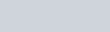# Why You Need A Compound Interest CalculatorA compound interest calculator computes the interest that your savings or investment has earned using the compounding interest. In order to understand the compounding interest calculator, we first have to understand what is Compound interest?

## Compound Interest and How It’s Calculated

You must have heard of the term compound interest all the time however confused on what it really means. Basically, a compound interest is an interest on the starting principal and on its accumulated interest. It’s an interest on an interest. Compound interest can be applied on loans and on investments too.

### How to use the Compound Interest Calculator

The compound interest calculator follows the compound interest formula. The formula is a standard followed by all financial institutions using the compounded interest rate. You can come up with the right calculations without all the fuss of computing by using a compound interest calculator provided on specific websites (financing websites, bank websites, etc).

The use of the compound interest calculator will require three things – the principal amount, the interest rate in percentage, and the number of years or time periods. Simply enter the figures being asked for and the calculator will compute your compounded interest annually, monthly or daily. Behind the calculator is a formula that is standard in computing compound interest.

### The Compound Interest FormulaWhere the values stand for the following:
‘A’ – the final amount of your investment
‘P’ – principal amount, starting capital
‘r’ – rate of the interest rate converted from percentage to decimal figures. In other formulation ‘r’ is interchangeable with ‘i’
‘n’ – number of time periods

#### Sample Scenario: Annual Compounding

If you have invested \$5,000 in savings time deposit with an interest of 5% annually for a period of two years compounding yearly, expect to receive \$512.50 on top of the principal amount.

A = \$5,000 x (1.05)2
A = \$5,000 x 1.1025
A = \$5,512.50

#### Sample Scenario: Monthly Compounding

If you have invested \$5,000 in savings time deposit with an interest of 5% annually for a period of two years compounding monthly. What is your expected returns on interests?

You will first need to get the interest monthly. To do that, divide the annual interest rate which is 5% or .05 by 12 (months). This will give you .42%

Then calculate the time period to months where to years is equivalent to 24 months.

Note: Monthly compounding yields bigger compared to annual compounding. In this scenario, the yield is at 550 which is greater than 512.50 yield in annual compounding.

A = \$5,000 x (1.0042)24
A = \$5,000 x 1.11
A = \$5,550

Investments computed on compounding interest provides more returns to the investor as compared to a simple interest rate. Using a compound interest calculator simplifies the process of computations so that you can focus more on deciding which investments will work for you best.

1 Response
1.Having read this I thought it was extremely informative.

I appreciate you taking the time and energy to put this information together.

I once again find myself spending a lot of time both reading and commenting.

But so what, it was still worthwhile!